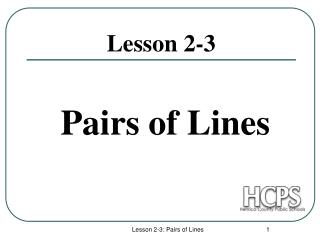DownloadDownload PresentationLesson 2-3

# Lesson 2-3

Télécharger la présentation## Lesson 2-3

- - - - - - - - - - - - - - - - - - - - - - - - - - - E N D - - - - - - - - - - - - - - - - - - - - - - - - - - -
##### Presentation Transcript

1. Pairs of Lines Lesson 2-3 Lesson 2-3: Pairs of Lines

2. Parallel Lines • Parallel lines are coplanar lines that do not intersect. • Arrows are used to indicate lines are parallel. • The symbol used for parallel lines is ||. In the above figure, the arrows show that line AB is parallel to line CD. With symbols we denote, . Lesson 2-3: Pairs of Lines

3. m n PERPENDICULAR LINES • Perpendicular lines are lines that intersect to form a right angle. • The symbol used for perpendicular lines is  . • 4 right angles are formed. In this figure line m is perpendicular to line n. With symbols we denote, m n Lesson 2-3: Pairs of Lines

4. OBLIQUE LINES • Oblique lines are lines that intersect, but do NOT form a right angle. • m n  Lesson 2-3: Pairs of Lines

5. Skew Lines and Parallel Planes • Two lines are skew if they do not intersect and are not in the same plane (not coplanar). Ex: • All planes are either parallel or intersecting. Parallel planes are two planes that do not intersect. Ex: Plane ABC and Plane EFG Lesson 2-3: Pairs of Lines

6. Examples: • Name all segments that are parallel to • Name all segments that intersect • Name all segments that are skew to • Name all planes that are parallel to plane ABC. Answers: • Segments BC, FG, & EH. • Segments DH, DC, AE & AB. • Segments CG, BF, FE, & GH. • Plane FGH. Lesson 2-3: Pairs of Lines

7. Slope of Parallel and Perpendicular lines • The slope of the non vertical line through the points and is m = The slope of a vertical line is not defined. The slope of a horizontal line is zero. Two lines are parallel if and only if they have equal slopes. Two lines are perpendicular if and only if the product of their slopes is -1 (reciprocals and opposite signs). Lesson 2-3: Pairs of Lines

8. Examples: Find the slope of the line through the given points. • (-4, 7) and (3, 7) • (3, -1) and (3, 2) • (1, -4) and (2, 5) • (-2, 5) and (1, -1) Lesson 2-3: Pairs of Lines

9. Examples Any line parallel to a line with slope has slope _____. Any line perpendicular to a line with slope has slope ___. Any line parallel to a line with slope 0 has slope _____. Any line perpendicular to a line with undefined slope has slope. Any line parallel to a line with slope 2 has slope _____. 0 Zero Slope 2 Lesson 2-3: Pairs of Lines